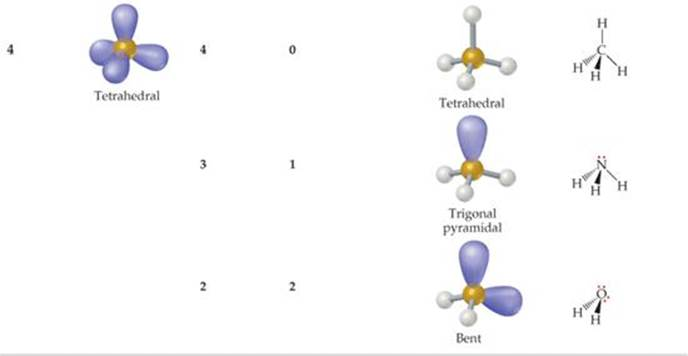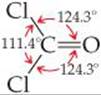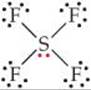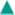﻿ ﻿THE VSEPR MODEL - MOLECULAR GEOMETRY AND BONDING THEORIES - CHEMISTRY THE CENTRAL SCIENCE

## 9 MOLECULAR GEOMETRY AND BONDING THEORIES

### 9.2 THE VSEPR MODEL

Imagine tying two identical balloons together at their ends. As shown inFIGURE 9.5, the two balloons naturally orient themselves to point away from each other; that is, they try to “get out of each other's way” as much as possible. If we add a third balloon, the balloons orient themselves toward the vertices of an equilateral triangle, and if we add a fourth balloon, they adopt a tetrahedral shape. We see that an optimum geometry exists for each number of balloons.

In some ways, the electrons in molecules behave like these balloons. We have seen that a single covalent bond is formed between two atoms when a pair of electrons occupies the space between the atoms.(Section 8.3) A bonding pair of electrons thus defines a region in which the electrons are most likely to be found. We will refer to such a region as an electron domain. Likewise, a nonbonding pair (or lone pair) of electrons defines an electron domain that is located principally on one atom. For example, the Lewis structure of NH3 has four electron domains around the central nitrogen atom (three bonding pairs, represented as usual by short lines, and one nonbonding pair, represented by dots):Each multiple bond in a molecule also constitutes a single electron domain. Thus, the resonance structure for O3 has three electron domains around the central oxygen atom (a single bond, a double bond, and a nonbonding pair of electrons):In general, each nonbonding pair, single bond, or multiple bond produces a single electron domain around the central atom in a molecule.GIVE IT SOME THOUGHT

Suppose a particular AB3 molecule has the resonance structureDoes this structure follow the octet rule? How many electron domains are there around the A atom?

TABLE 9.1 • Electron-Domain Geometries as a Function of Number of Electron DomainsThe VSEPR model is based on the idea that electron domains are negatively charged and therefore repel one another. Like the balloons in Figure 9.5, electron domains try to stay out of one another's way. The best arrangement of a given number of electron domains is the one that minimizes the repulsions among them. In fact, the analogy between electron domains and balloons is so close that the same preferred geometries are found in both cases. Like the balloons in Figure 9.5, two electron domains orient linearly, three domains orient in a trigonal-planar fashion, and four orient tetrahedrally. These arrangements, together with those for five- and six-electron domains, are summarized inTABLE 9.1. If you compare the geometries in Table 9.1 with those in Figure 9.3, you will see that they are the same. The shapes of different ABn molecules or ions depend on the number of electron domains surrounding the central atom.

The arrangement of electron domains about the central atom of an ABn molecule or ion is called its electron-domain geometry. In contrast, the molecular geometry is the arrangement of only the atoms in a molecule or ion—any nonbonding pairs in the molecule are not part of the description of the molecular geometry.

In determining the shape of any molecule, we first use the VSEPR model to predict the electron-domain geometry. From knowing how many of the domains are due to nonbonding pairs, we can then predict the molecular geometry. When all the electron domains in a molecule arise from bonds, the molecular geometry is identical to the electron-domain geometry. When, however, one or more domains involve nonbonding pairs of electrons, we must remember to ignore those domains when talking about molecular shape.

We can generalize the steps we follow in using the VSEPR model to predict the shapes of molecules or ions:

1. Draw the Lewis structure of the molecule or ion, and count the number of electron domains around the central atom. Each nonbonding electron pair, each single bond, each double bond, and each triple bond counts as one electron domain.

2. Determine the electron-domain geometry by arranging the electron domains about the central atom so that the repulsions among them are minimized, as shown in Table 9.1.

3. Use the arrangement of the bonded atoms to determine the molecular geometry.FIGURE 9.6 shows how these steps are applied to predict the geometry of the NH3 molecule. The three bonds and one nonbonding pair in the Lewis structure tell us we have four electron domains. We know from Table 9.1 that the repulsions among four electron domains are minimized when the domains point toward the vertices of a tetrahedron, so the electron-domain geometry of NH3 is tetrahedral. We know from the Lewis structure that one electron domain holds a nonbonding pair of electrons, which occupies one of the four vertices of the tetrahedron. The bonding arrangement is therefore three atoms bonded to a central atom, with the central atom not in the same plane as the three others. This is just the situation we find in the middle molecule of Figure 9.4. Hence, the molecular geometry of NH3 is trigonal pyramidal. Notice that the tetrahedral arrangement of the four electron domains leads us to predict the trigonal-pyramidal molecular geometry.

Because the trigonal-pyramidal molecular geometry is based on a tetrahedral electron-domain geometry, the ideal bond angles are 109.5°. As we will soon see, bond angles deviate from ideal values when the surrounding atoms and electron domains are not identical.GIVE IT SOME THOUGHT

From the standpoint of the VSEPR model, what do nonbonding electron pairs, single bonds, and multiple bonds have in common?FIGURE 9.6 Determining the molecular geometry of NH3.

As one more example, let's determine the shape of the CO2 molecule. Its Lewis structure reveals two electron domains (each one a double bond) around the central carbon:Two electron domains orient in a linear electron-domain geometry (Table 9.1). Because neither domain is a nonbonding pair of electrons, the molecular geometry is also linear, and the O—C—O bond angle is 180°.TABLE 9.2 summarizes the possible molecular geometries when an ABn molecule has four or fewer electron domains about A. These geometries are important because they include all the shapes usually seen in molecules or ions that obey the octet rule.

TABLE 9.2 • Electron-Domain and Molecular Geometries for Two, Three, and Four Electron Domains around a Central AtomSAMPLE EXERCISE 9.1 Using the VSEPR Model

Use the VSEPR model to predict the molecular geometry of (a) O3, (b) SnCl3.

SOLUTION

Analyze We are given the molecular formulas of a molecule and a polyatomic ion, both conforming to the general formula ABn and both having a central atom from the p block of the periodic table.

Plan To predict the molecular geometries, we draw their Lewis structures and count electron domains around the central atom to get the electron-domain geometry. We then obtain the molecular geometry from the arrangement of the domains that are due to bonds.

Solve

(a) We can draw two resonance structures for O3:Because of resonance, the bonds between the central O atom and the outer O atoms are of equal length. In both resonance structures the central O atom is bonded to the two outer O atoms and has one nonbonding pair. Thus, there are three electron domains about the central O atoms. (Remember that a double bond counts as a single electron domain.) The arrangement of three electron domains is trigonal planar (Table 9.1). Two of the domains are from bonds, and one is due to a nonbonding pair. So, the molecule has a bent shape with an ideal bond angle of 120° (Table 9.2).Comment As this example illustrates, when a molecule exhibits resonance, any one of the resonance structures can be used to predict the molecular geometry.

(b) The Lewis structure for SnCl3 isThe central Sn atom is bonded to the three Cl atoms and has one non-bonding pair; thus, we have four electron domains, meaning tetrahedral electron-domain geometry (Table 9.1) with one vertex occupied by a nonbonding pair of electrons. Tetrahedral electron-domain geometry with three bonding and one nonbonding domains means the molecular geometry is trigonal pyramidal (Table 9.2).PRACTICE EXERCISE

Predict the electron-domain and molecular geometries for (a) SeCl2, (b) CO32–.

Answers: (a) tetrahedral, bent; (b) trigonal planar, trigonal planar

Effect of Nonbonding Electrons and Multiple Bonds on Bond Angles

We can refine the VSEPR model to explain slight distortions from the ideal geometries summarized in Table 9.2. For example, consider methane (CH4), ammonia (NH3), and water (H2O). All three have a tetrahedral electron-domain geometry, but their bond angles differ slightly:Notice that the bond angles decrease as the number of nonbonding electron pairs increases. A bonding pair of electrons is attracted by both nuclei of the bonded atoms, but a nonbonding pair is attracted primarily by only one nucleus. Because a nonbonding pair experiences less nuclear attraction, its electron domain is spread out more in space than is the electron domain for a bonding pair (FIGURE 9.7). Nonbonding electron pairs therefore take up more space than bonding pairs. As a result, electron domains for nonbonding electron pairs exert greater repulsive forces on adjacent electron domains and tend to compress bond angles.

Because multiple bonds contain a higher electronic-charge density than single bonds, multiple bonds also represent enlarged electron domains. Consider the Lewis structure of phosgene:Because three electron domains surround the central atom, we might expect a trigonal-planar geometry with 120° bond angles. The double bond, however, seems to act much like a nonbonding pair of electrons, reducing the Cl—C—Cl bond angle to 111.4°:In general, electron domains for multiple bonds exert a greater repulsive force on adjacent electron domains than do electron domains for single bonds.GIVE IT SOME THOUGHT

One resonance structure of the nitrate ion isThe bond angles in this ion are 120°. Is this observation consistent with the preceding discussion of the effect of multiple bonds on bond angles?

Molecules with Expanded Valence Shells

Atoms from period 3 and beyond may be surrounded by more than four electron pairs.(Section 8.7) Molecules with five or six electron domains around the central atom have molecular geometries based on either a trigonal-bipyramidal (five domains) or octahedral (six domains) electron-domain geometry (TABLE 9.3).

The most stable electron-domain geometry for five electron domains is the trigonal bipyramid (two trigonal pyramids sharing a base). Unlike the other arrangements we have seen, the electron domains in a trigonal bipyramid can point toward two geometrically distinct types of positions. Two domains point toward axial positions and three point toward equatorial positions (FIGURE 9.8). Each axial domain makes a 90° angle with any equatorial domain. Each equatorial domain makes a 120° angle with either of the other two equatorial domains and a 90° angle with either axial domain.

Suppose a molecule has five electron domains, and there are one or more nonbonding pairs. Will the domains from the nonbonding pairs occupy axial or equatorial positions? To answer this question, we must determine which location minimizes repulsion between domains. Repulsion between two domains is much greater when they are situated 90° from each other than when they are at 120°. An equatorial domain is 90° from only two other domains (the axial domains), but an axial domain is 90° from three other domains (the equatorial domains). Hence, an equatorial domain experiences less repulsion than an axial domain. Because the domains from nonbonding pairs exert larger repulsions than those from bonding pairs, nonbonding domains always occupy the equatorial positions in a trigonal bipyramid.GIVE IT SOME THOUGHT

It might seem that a square-planar geometry of four electron domains around a central atom would be more favorable than a tetrahedron. Can you rationalize why the tetrahedron is preferred, based on angles between electron domains?GO FIGURE

Why is the volume occupied by the nonbonding electron pair domain larger than the volume occupied by the bonding domain?FIGURE 9.7 Relative volumes occupied by bonding and nonbonding electron domains.GO FIGURE

What is the bond angle formed by an axial atom, the central atom, and any equatorial atom?FIGURE 9.8 In a trigonal-bipyramidal geometry, there are two types of positions for the outer atoms.

TABLE 9.3 • Electron-Domain and Molecular Geometries for Five and Six Electron Domains around a Central AtomThe most stable electron-domain geometry for six electron domains is the octahedron. An octahedron is a polyhedron with six vertices and eight faces, each an equilateral triangle. An atom with six electron domains around it can be visualized as being at the center of the octahedron with the electron domains pointing toward the six vertices, as shown in Table 9.3. All the bond angles are 90°, and all six vertices are equivalent. Therefore, if an atom has five bonding electron domains and one nonbonding domain, we can put the nonbonding domain at any of the six vertices of the octahedron. The result is always a square-pyramidal molecular geometry. When there are two non-bonding electron domains, however, their repulsions are minimized by pointing them toward opposite sides of the octahedron, producing a square-planar molecular geometry, as shown in Table 9.3.

SAMPLE EXERCISE 9.2 Molecular Geometries of Molecules with Expanded Valence Shells

Use the VSEPR model to predict the molecular geometry of (a) SF4, (b) IF5.

SOLUTION

Analyze The molecules are of the ABn type with a central p-block atom.

Plan We first draw Lewis structures and then use the VSEPR model to determine the electron-domain geometry and molecular geometry.

Solve

(a) The Lewis structure for SF4 isThe sulfur has five electron domains around it: four from the S—F bonds and one from the nonbonding pair. Each domain points toward a vertex of a trigonal bipyramid. The domain from the nonbonding pair will point toward an equatorial position. The four bonds point toward the remaining four positions, resulting in a molecular geometry that is described as seesaw-shaped:Comment The experimentally observed structure is shown on the right. We can infer that the nonbonding electron domain occupies an equatorial position, as predicted. The axial and equatorial S—F bonds are slightly bent away from the nonbonding domain, suggesting that the bonding domains are “pushed” by the nonbonding domain, which exerts a greater repulsion (Figure 9.7).

(b) The Lewis structure of IF5 isThe iodine has six electron domains around it, one of which is nonbonding. The electron-domain geometry is therefore octahedral, with one position occupied by the nonbonding pair, and the molecular geometry is square pyramidal (Table 9.3):Comment Because the nonbonding domain is larger than the bonding domains, we predict that the four F atoms in the base of the pyramid will be tipped up slightly toward the top F atom. Experimentally, we find that the angle between the base atoms and top F atom is 82°, smaller than the ideal 90° angle of an octahedron.

PRACTICE EXERCISE

Predict the electron-domain and molecular geometries of (a) BrF3, (b) ICl4.

Answers: (a) trigonal bipyramidal, T-shaped; (b) octahedral, square planarGO FIGURE

Although the electron-domain geometry around the right O is tetrahedral, the C—O—H bond is slightly less than 109.5o. Explain.FIGURE 9.9 Acetic acid, CH3COOH.

Shapes of Larger Molecules

Although the molecules and ions we have considered contain only a single central atom, the VSEPR model can be extended to more complex molecules. For the acetic acid molecule, for example,we can use the VSEPR model to predict the geometry about each atom:The left C has four electron domains (all bonding), and so the electron-domain and molecular geometries around that atom are both tetrahedral. The central C has three electron domains (counting the double bond as one domain), making both the electron-domain and the molecular geometries trigonal planar. The O on the right has four electron domains (two bonding, two nonbonding), so its electron-domain geometry is tetrahedral and its molecular geometry is bent. The bond angles about the central C atom and the O atom are expected to deviate slightly from the ideal values of 120° and 109.5° because of the spatial demands of multiple bonds and nonbonding electron pairs.

The structure of the acetic acid molecule is shown inFIGURE 9.9.

SAMPLE EXERCISE 9.3 Predicting Bond Angles

Eyedrops for dry eyes usually contain a water-soluble polymer called poly(vinyl alcohol), which is based on the unstable organic molecule vinyl alcohol:Predict the approximate values for the H—O—C and O—C—C bond angles in vinyl alcohol.

SOLUTION

Analyze We are given a Lewis structure and asked to determine two bond angles.

Plan To predict a bond angle, we determine the number of electron domains surrounding the middle atom in the bond. The ideal angle corresponds to the electron-domain geometry around the atom. The angle will be compressed somewhat by nonbonding electrons or multiple bonds.

Solve In H—O—C, the O atom has four electron domains (two bonding, two nonbonding). The electron-domain geometry around O is therefore tetrahedral, which gives an ideal angle of 109.5°. The H—O—C angle is compressed somewhat by the nonbonding pairs, so we expect this angle to be slightly less than 109.5°.

To predict the O—C—C bond angle, we examine the middle atom in the angle. In the molecule, there are three atoms bonded to this C atom and no nonbonding pairs, and so it has three electron domains about it. The predicted electron-domain geometry is trigonal planar, resulting in an ideal bond angle of 120°. Because of the larger size of the C═C domain, the bond angle should be slightly greater than 120°.

PRACTICE EXERCISE

Predict the H—C—H and C—C—C bond angles in propyne: